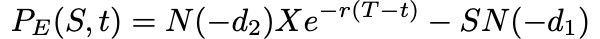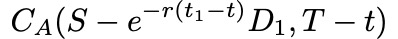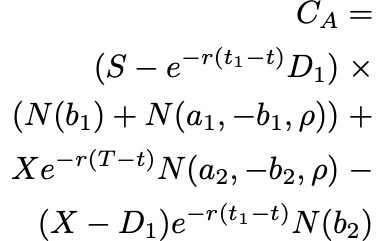# The Black-Scholes formula, explained

##### Share:

Very informally, the two terms in the sum given by the Black-Scholes formula may be thought of as ‘the current price of the stock weighted by the probability that you will exercise your option to buy the stock’ minus ‘the discounted price of exercising the option weighted by the probability that you will exercise the option’, or simply ‘what you are going to get’ minus ‘what you are going to pay’ (Khan, 2013).

For a European put option (contracts for the right, but not obligation, to sell some underlaying asset at a pre-determined price on a pre-determined date in the future) the equivalent functional form is:Equation 9. The Black-Scholes formula for the value of a put option C for a non-dividend paying stock of price S

## Example: Calculating the price of a European call option

In order to calculate what the price of a European call option should be, we know we need five values required by equation 6 above. They are: 1. The current price of the stock (S), 2. The exercise price of the call option (X), 3. The time to expiration (T - t), 4. The risk-free interest rate (r) and 5. The volatility of the stock, given by the standard deviation of historical log returns (σ).

`Estimating the value of a call option for Tesla (TSLA)The first four values we need are easily obtainable. Let’s say we are interested in a call option for Tesla’s stock (\$TSLA), maturing the day of its Q3 earnings in 2019, at a price 20% higher than the stock is currently trading. Looking at Tesla’s NASDAQ listing (\$TSLA) on Yahoo Finance today (July 13th, 2019), we find a stock price of S = \$245. Multiplying the current price with 1.2 gives us an exercise price 20% higher than the stock is currently trading, X = \$294. Googling, we find that the day of its Q3 earnings call is October 22nd, giving us a time to expiration/maturity of Oct 22nd - July 13th = 101 days. As a proxy for a risk-free interest rate instrument, we’ll use US 10-year government bonds (\$USGG10YR), currently paying off 2.12%.So, we find S = 245, X = 294, T - t = 101 and r = 0.0212. The only missing value is an estimation of the stock’s volatility (σ).`

We can estimate any stock’s volatility by observing its historical prices, or, even simpler, by calculating other option prices for the same stock at different maturity/expiration dates (T) and exercise/strike prices (X), if we know they have been set according to a Black-Scholes model. The resulting value, σ, is a number between 0 and 1, representing the market’s implied volatility for the stock. For Tesla, at the time of writing this article, the value averaged at approximately 0.38 for 4–5 different option prices around the same expiry/maturity date. Input into equation 6 above, we find that the call option we’re interested in should be priced somewhere around \$7.

## Implied volatility

Although it is interesting to understand how options issuers arrive at the price of their call and put options, as investors it’s hard to “disagree” with such prices, per se, and so difficult to turn this knowledge into actionable investment theses.

We can however get a lot of milage out of the Black-Scholes formula if we instead treat the price of an option (C or P) as a known quantity/independent variable (found by looking at different maturity/expiration dates T and different exercise prices X). This because, if we do, the Black-Scholes functional equation becomes a tool to help us understand how the market estimates the volatility of a stock, also known as the implied volatility of the option. This is information we can disagree over, and trade against.

`Hypothetical scenarioIf we for instance look at the chart for the Tesla stock over the last three months (figure 2), we see a rather (for a lack of a better word) volatile journey from hovering around \$280 three months ago, to a low of \$180 a month and a half ago, to now on its way back up at \$245. This makes sense given the volatility we observed from call prices before (\$280–\$180 = \$100, \$100/280 = 0.36, vs 0.38). It does not make sense, however, if we think the fluctuation over the past three months was the mere tip of an iceberg, going into a period of more volatility for Tesla, say, due to an upcoming increase in short-selling.`
`Let's say we disagree with an options issuer about the implied volatility of stock's performance over the last three months. We think the ride is going to get rockier. How much? Let's say that instead of 40%, we think the next three months will look more like 60%. Input into the functional Black-Scholes formula along with the same values for S, X, r, and T - t, we get a price of nearly twice of what the options issuer wants, at C(S,t) = \$14.32. This we can trade on. We could, for instance, buy call options today and wait for volatility to increase or the value of the stock to go up, before selling at a profit.`

# American options

Because American options can be exercised at any date prior to expiration (so-called “continuous timeline instruments”), they are much more difficult to deal with that European options (“point in time instruments”). Primarily, since the optimal exercise policy will affect the value of the option, this needs to be taken into account when solving the Black-Scholes partial differential equation. There are no known “closed form” solutions for American options according to the Black-Scholes equation. There are, though, some special cases:

• For American call options on underlying assets that do not pay dividend (or other payouts), the American call option price is the same as for European call options. This because the optimal exercise policy in this case is to not exercise the option.
• For American call options on underlying assets that do pay one known dividend in its lifetime, it may be optimal to exercise the option early. In such cases the option may be optimally exercised just before the stock goes ex-dividend, according to a solution given in closed-form by the so-called Roll-Geske-Whaley method (Roll, 1977; Geske, 1979; 1981; Whaley, 1981):

First, check if it is optimal to exercise the option early, by investigating whether the following inequality is fulfilled:

For S = stock price, X = exercise price, D₁ = dividend paid, t = current date, t₁ = date of dividend payment, T = expiration date of option.

If the inequality is not fulfilled, early exercise it not optimal. If C(・) is the regular Black-Scholes formula for European call options on non-dividend-paying stock (eq x), the value of the American call option is then given by a version of the same equation where the stock price (S) is discounted:Equation 11. The value of an American call option when inequality (eq.8) is not fulfilled

If the inequality is fulfilled, early exercise is optimal and the value of the American call option is given by the following, awful, mess of an equation (I tried to break it up by each term to make it more readable):Equation 12. The value of an American call option when inequality (eq. 10) is fulfilled

Whereas before S = price of stock, T = date of expiration of option, X = exercise price and r = risk-free interest rate, σ = volatility (standard deviation of the log of the historical returns of the stock), and D₁ is the dividend payout. In addition, ρ is given by:

a₁, a₂ by:

and b₁, b₂ by:

# Limitations

It should go without saying that Black-Scholes model is precisely that, a theoretical model that tries to estimate how a market behaves, given the assumptions stated above and the inherent limitations of our own numerical estimations of risk-free interest rates (r) and future volatility (σ). It should here be highlighted that not all the assumptions of (especially the original model) are in fact empirically valid. For instance, significant limitations arise from:

• The underestimation of extreme moves in the stock, yielding tail risk
• The assumption of instant, cost-less trading, yielding liquidity risk
• The assumption of a stationary process, yielding volatility risk
• The assumption of continuous time and trading, yielding gap risk

These should be accounted for in any and all investment strategies, for instance by hedging with out-of-the-money options, trading on multiple exchanges, hedging with volatility hedging and Gamma hedging, respectively.

# Background

As briefly mentioned it was Fischer Black and Myron Scholes who in 1973 showed that dynamically revising a portfolio according to certain rules removes the expected return of the underlying security (Black & Scholes, 1973). Their model built on previously established works by Bachelier, Samuelson and others. Robert C. Merton was the first to publish a paper expanding on the understanding of the model and who coined the term “Black-Scholes options pricing model”. Scholes and Merton was awarded the 1997 Nobel Memorial Prize in Economic Sciences for their discovery of the method of divorcing stock options from the risk of their underlying securities. As Fischer Black passed away in 1995, he would not be eligible to receive the award, but was acknowledged as a contributor by the Nobel Academy.